# 1.17. Neural network models (supervised)¶

Warning

This implementation is not intended for large-scale applications. In particular, scikit-learn offers no GPU support. For much faster, GPU-based implementations, as well as frameworks offering much more flexibility to build deep learning architectures, see Related Projects.

## 1.17.1. Multi-layer Perceptron¶

Multi-layer Perceptron (MLP) is a supervised learning algorithm that learns a function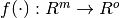by training on a dataset, where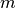is the number of dimensions for input and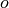is the number of dimensions for output. Given a set of features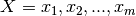and a target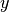, it can learn a non-linear function approximator for either classification or regression. It is different from logistic regression, in that between the input and the output layer, there can be one or more non-linear layers, called hidden layers. Figure 1 shows a one hidden layer MLP with scalar output.

The leftmost layer, known as the input layer, consists of a set of neurons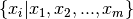representing the input features. Each neuron in the hidden layer transforms the values from the previous layer with a weighted linear summation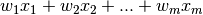, followed by a non-linear activation function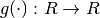- like the hyperbolic tan function. The output layer receives the values from the last hidden layer and transforms them into output values.

The module contains the public attributes coefs_ and intercepts_. coefs_ is a list of weight matrices, where weight matrix at indexrepresents the weights between layerand layer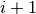. intercepts_ is a list of bias vectors, where the vector at indexrepresents the bias values added to layer.

The advantages of Multi-layer Perceptron are:

• Capability to learn non-linear models.
• Capability to learn models in real-time (on-line learning) using partial_fit.

The disadvantages of Multi-layer Perceptron (MLP) include:

• MLP with hidden layers have a non-convex loss function where there exists more than one local minimum. Therefore different random weight initializations can lead to different validation accuracy.
• MLP requires tuning a number of hyperparameters such as the number of hidden neurons, layers, and iterations.
• MLP is sensitive to feature scaling.

## 1.17.2. Classification¶

Class MLPClassifier implements a multi-layer perceptron (MLP) algorithm that trains using Backpropagation.

MLP trains on two arrays: array X of size (n_samples, n_features), which holds the training samples represented as floating point feature vectors; and array y of size (n_samples,), which holds the target values (class labels) for the training samples:

>>> from sklearn.neural_network import MLPClassifier
>>> X = [[0., 0.], [1., 1.]]
>>> y = [0, 1]
>>> clf = MLPClassifier(solver='lbfgs', alpha=1e-5,
...                     hidden_layer_sizes=(5, 2), random_state=1)
...
>>> clf.fit(X, y)
MLPClassifier(activation='relu', alpha=1e-05, batch_size='auto',
beta_1=0.9, beta_2=0.999, early_stopping=False,
epsilon=1e-08, hidden_layer_sizes=(5, 2), learning_rate='constant',
learning_rate_init=0.001, max_iter=200, momentum=0.9,
nesterovs_momentum=True, power_t=0.5, random_state=1, shuffle=True,
solver='lbfgs', tol=0.0001, validation_fraction=0.1, verbose=False,
warm_start=False)


After fitting (training), the model can predict labels for new samples:

>>> clf.predict([[2., 2.], [-1., -2.]])
array([1, 0])


MLP can fit a non-linear model to the training data. clf.coefs_ contains the weight matrices that constitute the model parameters:

>>> [coef.shape for coef in clf.coefs_]
[(2, 5), (5, 2), (2, 1)]


Currently, MLPClassifier supports only the Cross-Entropy loss function, which allows probability estimates by running the predict_proba method.

MLP trains using Backpropagation. More precisely, it trains using some form of gradient descent and the gradients are calculated using Backpropagation. For classification, it minimizes the Cross-Entropy loss function, giving a vector of probability estimates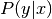per sample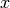:

>>> clf.predict_proba([[2., 2.], [1., 2.]])
array([[  1.967...e-04,   9.998...-01],
[  1.967...e-04,   9.998...-01]])


MLPClassifier supports multi-class classification by applying Softmax as the output function.

Further, the model supports multi-label classification in which a sample can belong to more than one class. For each class, the raw output passes through the logistic function. Values larger or equal to 0.5 are rounded to 1, otherwise to 0. For a predicted output of a sample, the indices where the value is 1 represents the assigned classes of that sample:

>>> X = [[0., 0.], [1., 1.]]
>>> y = [[0, 1], [1, 1]]
>>> clf = MLPClassifier(solver='lbfgs', alpha=1e-5,
...                     hidden_layer_sizes=(15,), random_state=1)
...
>>> clf.fit(X, y)
MLPClassifier(activation='relu', alpha=1e-05, batch_size='auto',
beta_1=0.9, beta_2=0.999, early_stopping=False,
epsilon=1e-08, hidden_layer_sizes=(15,), learning_rate='constant',
learning_rate_init=0.001, max_iter=200, momentum=0.9,
nesterovs_momentum=True, power_t=0.5, random_state=1, shuffle=True,
solver='lbfgs', tol=0.0001, validation_fraction=0.1, verbose=False,
warm_start=False)
>>> clf.predict([[1., 2.]])
array([[1, 1]])
>>> clf.predict([[0., 0.]])
array([[0, 1]])


See the examples below and the doc string of MLPClassifier.fit for further information.

## 1.17.3. Regression¶

Class MLPRegressor implements a multi-layer perceptron (MLP) that trains using backpropagation with no activation function in the output layer, which can also be seen as using the identity function as activation function. Therefore, it uses the square error as the loss function, and the output is a set of continuous values.

MLPRegressor also supports multi-output regression, in which a sample can have more than one target.

## 1.17.4. Regularization¶

Both MLPRegressor and class:MLPClassifier use parameter alpha for regularization (L2 regularization) term which helps in avoiding overfitting by penalizing weights with large magnitudes. Following plot displays varying decision function with value of alpha.

See the examples below for further information.

## 1.17.5. Algorithms¶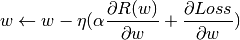where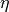is the learning rate which controls the step-size in the parameter space search.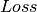is the loss function used for the network.

More details can be found in the documentation of SGD

Adam is similar to SGD in a sense that it is a stochastic optimizer, but it can automatically adjust the amount to update parameters based on adaptive estimates of lower-order moments.

With SGD or Adam, training supports online and mini-batch learning.

L-BFGS is a solver that approximates the Hessian matrix which represents the second-order partial derivative of a function. Further it approximates the inverse of the Hessian matrix to perform parameter updates. The implementation uses the Scipy version of L-BFGS.

If the selected solver is ‘L-BFGS’, training does not support online nor mini-batch learning.

## 1.17.6. Complexity¶

Suppose there are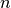training samples,features,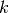hidden layers, each containing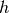neurons - for simplicity, andoutput neurons. The time complexity of backpropagation is, whereis the number of iterations. Since backpropagation has a high time complexity, it is advisable to start with smaller number of hidden neurons and few hidden layers for training.

## 1.17.7. Mathematical formulation¶

Given a set of training examples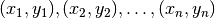where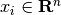and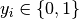, a one hidden layer one hidden neuron MLP learns the function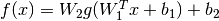where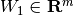and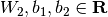are model parameters.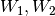represent the weights of the input layer and hidden layer, resepctively; and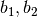represent the bias added to the hidden layer and the output layer, respectively.is the activation function, set by default as the hyperbolic tan. It is given as,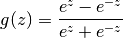For binary classification,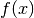passes through the logistic function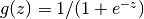to obtain output values between zero and one. A threshold, set to 0.5, would assign samples of outputs larger or equal 0.5 to the positive class, and the rest to the negative class.

If there are more than two classes,itself would be a vector of size (n_classes,). Instead of passing through logistic function, it passes through the softmax function, which is written as,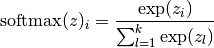where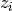represents theth element of the input to softmax, which corresponds to class, and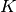is the number of classes. The result is a vector containing the probabilities that samplebelong to each class. The output is the class with the highest probability.

In regression, the output remains as; therefore, output activation function is just the identity function.

MLP uses different loss functions depending on the problem type. The loss function for classification is Cross-Entropy, which in binary case is given as,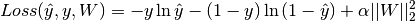where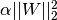is an L2-regularization term (aka penalty) that penalizes complex models; and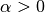is a non-negative hyperparameter that controls the magnitude of the penalty.

For regression, MLP uses the Square Error loss function; written as,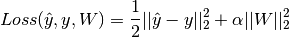Starting from initial random weights, multi-layer perceptron (MLP) minimizes the loss function by repeatedly updating these weights. After computing the loss, a backward pass propagates it from the output layer to the previous layers, providing each weight parameter with an update value meant to decrease the loss.

In gradient descent, the gradient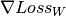of the loss with respect to the weights is computed and deducted from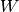. More formally, this is expressed as,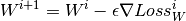whereis the iteration step, andis the learning rate with a value larger than 0.

The algorithm stops when it reaches a preset maximum number of iterations; or when the improvement in loss is below a certain, small number.

## 1.17.8. Tips on Practical Use¶

• Multi-layer Perceptron is sensitive to feature scaling, so it is highly recommended to scale your data. For example, scale each attribute on the input vector X to [0, 1] or [-1, +1], or standardize it to have mean 0 and variance 1. Note that you must apply the same scaling to the test set for meaningful results. You can use StandardScaler for standardization.

>>> from sklearn.preprocessing import StandardScaler
>>> scaler = StandardScaler()
>>> # Don't cheat - fit only on training data
>>> scaler.fit(X_train)
>>> X_train = scaler.transform(X_train)
>>> # apply same transformation to test data
>>> X_test = scaler.transform(X_test)


An alternative and recommended approach is to use StandardScaler in a Pipeline

• Finding a reasonable regularization parameter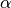is best done using GridSearchCV, usually in the range 10.0 ** -np.arange(1, 7).

• Empirically, we observed that L-BFGS converges faster and with better solutions on small datasets. For relatively large datasets, however, Adam is very robust. It usually converges quickly and gives pretty good performance. SGD with momentum or nesterov’s momentum, on the other hand, can perform better than those two algorithms if learning rate is correctly tuned.

## 1.17.9. More control with warm_start¶

If you want more control over stopping criteria or learning rate in SGD, or want to do additional monitoring, using warm_start=True and max_iter=1 and iterating yourself can be helpful:

>>> X = [[0., 0.], [1., 1.]]
>>> y = [0, 1]
>>> clf = MLPClassifier(hidden_layer_sizes=(15,), random_state=1, max_iter=1, warm_start=True)
>>> for i in range(10):
...     clf.fit(X, y)
...     # additional monitoring / inspection
MLPClassifier(...


References: